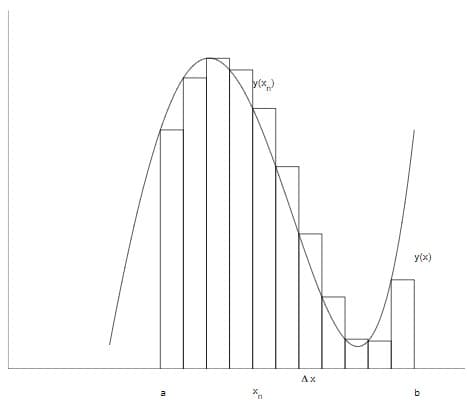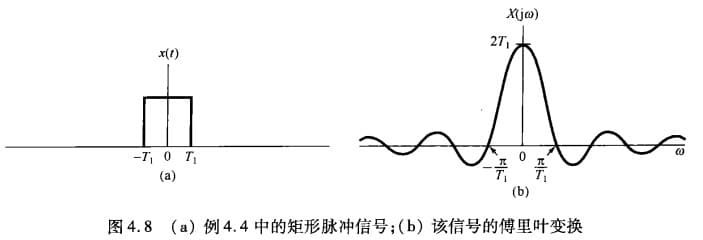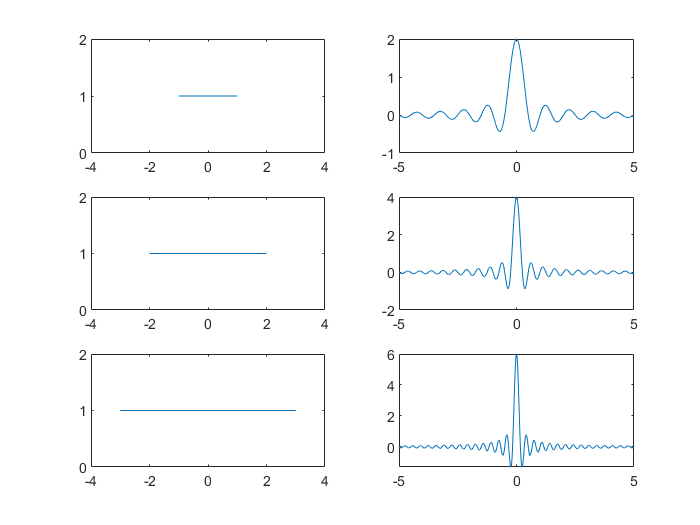# 连续时间傅里叶变换

\begin{align*} \newcommand{\dif}{\mathop{}\!\mathrm{d}} \newcommand{\xleftrightarrow}{\stackrel{#1}{\longleftrightarrow}} \end{align*}

# 连续傅里叶变换

## 引入

1. $\widetilde{x}(t)$ 是周期的，并且周期为 $2L$
2. $L$ 很大
3. $\widetilde{x}(t)$ 和 $x(t)$ 在 $-L<t<L$ 上具有相同值

$\Delta\omega$ 表示相邻 $\omega_k$ 间的距离。代入傅里叶展式：# 收敛条件

The Fourier integral transform of the function $h(t)$,

will exist if either

Dirichlet Conditions in Fourier Transformation are as follows:

1. f(x) must absolutely integrable over a period（绝对可积）, which is:

2. f(x) must have a finite number of exterma in any given interval, i.e. there must be a finite number of maxima and minima in the interval（有限区间内最值有限）.
3. f(x) must have a finite number of discontinues in any given interval, however the discontinuity cannot be infinite.（有限区间内有有限第一间断点）

# 常见信号的傅里叶变换

## 矩形脉冲信号t=-1:.01:1; %T1
k=0;
for f=-5:.01:5
k=k+1;
X(k)=trapz(t, exp(-j*2*pi*f*t)); %傅里叶变换
end
f=-5:.01:5;
plot(f,X)